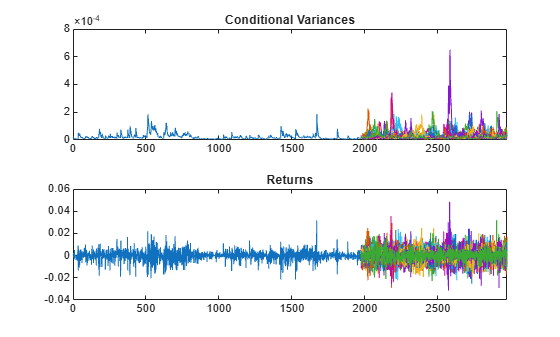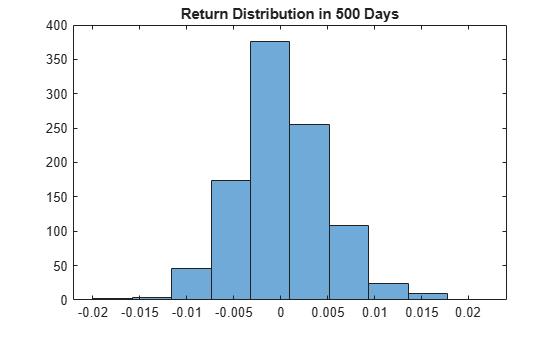# Simulate Conditional Variance Model

This example shows how to simulate a conditional variance model using `simulate`.

### Step 1. Load the data and specify the model.

Load the Deutschmark/British pound foreign exchange rate data included with the toolbox, and convert to returns. Specify and fit a GARCH(1,1) model.

```load Data_MarkPound r = price2ret(Data); T = length(r); Mdl = garch(1,1); EstMdl = estimate(Mdl,r);```
``` GARCH(1,1) Conditional Variance Model (Gaussian Distribution): Value StandardError TStatistic PValue __________ _____________ __________ __________ Constant 1.0535e-06 3.5048e-07 3.0058 0.0026487 GARCH{1} 0.80657 0.01291 62.478 0 ARCH{1} 0.15436 0.011574 13.336 1.4293e-40 ```
`v0 = infer(EstMdl,r);`

### Step 2. Simulate foreign exchange rate returns.

Use the fitted model to simulate 25 realizations of foreign exchange rate returns and conditional variances over a 1000-period forecast horizon. Use the observed returns and inferred conditional variances as presample innovations and variances, respectively.

```rng default; % For reproducibility [V,Y] = simulate(EstMdl,1000,'NumPaths',25,... 'E0',r,'V0',v0); figure subplot(2,1,1) plot(v0) hold on plot(T+1:T+1000,V) xlim([0,T+1000]) title('Conditional Variances') hold off subplot(2,1,2) plot(r) hold on plot(T+1:T+1000,Y) xlim([0,T+1000]) title('Returns') hold off```### Step 3. Plot the returns distribution at a future time.

Use simulations to generate a forecast distribution of foreign exchange returns 500 days into the future. Generate 1000 sample paths to estimate the distribution.

```rng default; % For reproducibility [V,Y] = simulate(EstMdl,500,'NumPaths',1000,... 'E0',r-EstMdl.Offset,'V0',v0); figure histogram(Y(500,:),10) title('Return Distribution in 500 Days')```